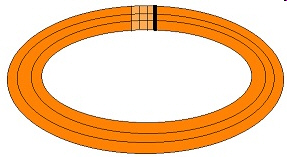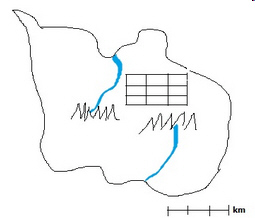3.MD.6.-Test1B Measure areas by counting unit squares
 Name:    3.MD.6.-Test1B Measure areas by counting unit squares

Multiple Choice
Identify the choice that best completes the statement or answers the question.

1.

What is the symbol for items measured in meters?
 a. 1n c. m b. cm d. km

2.

What is the symbol used for something measuring kilometers?
 a. in c. m b. cm d. km

3.

What symbol is used for something being measured in inches?
 a. in c. m b. cm d. km

4.

What symbol is used to measure an object in centimeters?
 a. in c. m b. cm d. km

5.

Area is measured in ___________________ units.
 a. triangular c. square b. circular d. rectangular

6.

Running tracks are divided into 400 meters.  Each lane is about 1 meter wide.  What is the area of the light brown area of this track?a. 9 sq in c. 9 sq km b. 9 sq m d. 9 sq cm

7.

What is the area of the section outlined in squares on the map?a. 15 sq km c. 18 sq km b. 12 sq km d. 9 sq km

8.

What is the area of the of the front of the square blue box?a. 9 sq cm c. 9 sq m b. 9 sq in d. 9 sq km

9.

What is the area of the front of this square box?a. 9 sq cm c. 9 sq m b. 9 sq in d. 9 sq km